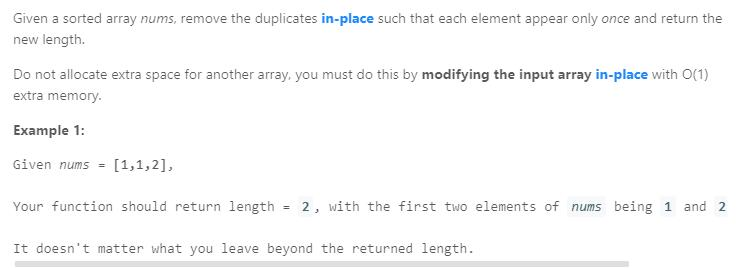# 题目描述（简单难度）# 我写的

for 循环遍历每个数，while 循环判断当前数和它的后一个数是否相等，相等就后移一个数，并且接着判断后移的数和它后边的数是否相等，然后一直循环下去。不相等就将后一个数保存起来，并且长度加 1，然后结束循环。

public int removeDuplicates(int[] nums) {
int len = 1;
for (int i = 0; i < nums.length - 1; i++) {
while (i < nums.length - 1) {
if (nums[i] == nums[i + 1]) {
i++;
} else {
nums[len] = nums[i + 1];
len = len + 1;
break;
}
}
}
return len;
}


# Solution 给出的

public int removeDuplicates(int[] nums) {
if (nums.length == 0) return 0;
int i = 0;
for (int j = 1; j < nums.length; j++) {
if (nums[j] != nums[i]) {
i++;
nums[i] = nums[j];
}
}
return i + 1;
}